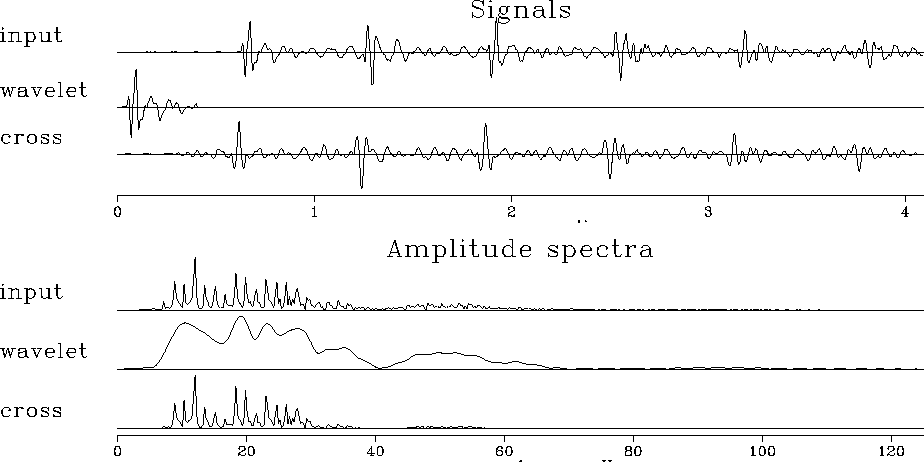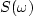## Matched filtering

Figure 13 shows a deep-water seismogram where the bottom is unusually hard. The second signal is the wavelet that results from windowing about the first water-bottom reflection. Notice that the wavelet has a comparatively simple spectrum, its principal feature being that it vanishes at low frequencies and high frequencies. The input has a spectrum that is like that of the wavelet, but multiplied by a fine-toothed comb reminiscent of cmb5'' in Figure 12.match
Figure 13
Example of matched filtering with water-bottom reflection. Top shows signals and bottom shows corresponding spectra. The result was time shifted to best align with the input.

Matched filtering''

is crosscorrelating with a wavelet. Equivalently, it is convolving with the time-reversed wavelet. Matched filtering uses Y(Z)=F(1/Z)X(Z) instead of Y(Z)=F(Z)X(Z). The third signal in Figure 13 shows the data crosscorrelated with the sea-floor reflection. Notice that the output sea-floor reflection is symmetric like an autocorrelation function. Later bounces are crosscorrelations, but they resemble the autocorrelation. Ideally, alternate water-bottom reflections have alternating polarities. From the figure you can see that matched filtering makes this idealization more apparent. An annoying feature of the matched filter is that it is noncausal, i.e., there is an output before there is an input. You can see this in Figure 13 just before the water-bottom reflection.

## EXERCISES:

1. Suppose a wavelet is made up of complex numbers. Is the autocorrelation relation sk = s-k true? Is sk real or complex? Isreal or complex?
2. If concepts of time and frequency are interchanged, what does the meaning of spectrum become?
3. Suggest a reason why the spectrum of the wavelet in Figure 13 contains more low-frequency energy than the whole seismogram.
4. Suggest a reason why the spectrum of the wavelet in Figure 13 contains more high-frequency energy than the whole seismogram.